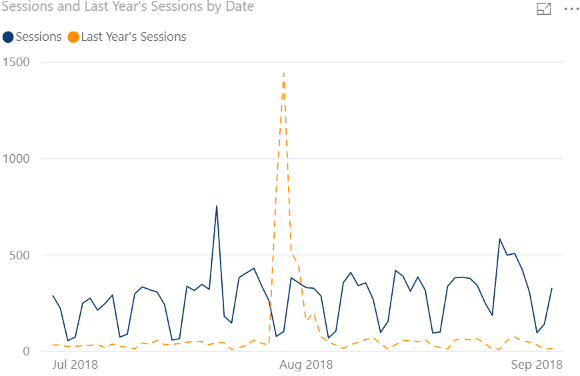# How to Use CALCULATE in Power BI

## Great Uses of CALCULATE in Power BI

Calculate is one of the most versatile functions in Power BI. When you begin using anything from simple filters, time intelligence functions, or even advanced formulas, often the CALCULATE formulas are leveraged to produce the desired outcome.

Let’s use CALCULATE to filter a column in a table. CALCULATE can be used for single filter conditions or multiple filter conditions. Let’s explore the function’s syntax. If you are familiar with Tableau, the equivalent would be the level of detail functions.

### How to Use Calculate

CALCULATE(Expression, Filter1)

Quick Rules for CALCULATE.

The filter section of calculate can only be:

• a number
•  text
• a date

In the formula, there are two basic arguments. The expression and the filter. An expression can be anything from an aggregation or calculated measure. For these purposes, we are going to use the SUM and Average functions with our CALCULATE function.  However, please remember you are not limited to these aggregations.

CALCULATE(SUM(Table[Column],Filter1)CALCULATE(SUM(Table[Column],Filter1)CALCULATE(AVERAGE(Website[Sessions]),Website[type]=”Organic Search”)

The benefit of using CALCULATE to filter is using your newly created calculated measures to add a layer of context and complexity to your visualizations. For example, overlaying the amount of website organic search visits to the total am your visuals. This can be achieved with the intricate filter on your visualization pane, however, it can be done rather quickly using these CALCULATE measures. Usually, when your data is a column field, you are restricted to the stacked graphs and filter to isolate the dimension of choice. The CALCULATE function can help circumvent this.

### Use Calculate with Two or More Filters.

Sometimes your data may need more to be filtered by more than one condition to provide you with the segment that you are interested in.   Imagine you have the challenge of finding out the number of sessions for your organic search visits from females. This can be achieved by adding an additional filter to your CALCULATE function.

To use CALCULATE with multiple filters you can just simply add another filter condition.:

CALCULATE(AVERAGE(Website[Sessions]),Website[type]=”Organic Search”, Website[gender]=’female’)

You can have as many conditions as you want by adding more conditional filters. We will explore other conditions using some of the time intelligence functions in Power BI.### Use Calculate with Time Intelligence Functions

Calculate is a great function to compare with time intelligence functions such as SAMEPERIODLASTYEAR, PREVIOUS MONTH, PREVIOUSDAY.

For me, this is one of the best uses of CALCULATE to evaluate time-series data. Let’s look at the example below which will compare prices this year versus last year.

** A lot of time intelligence functions will not function if you don’t have continuous dates in your table.

The formula we are using is below:

CALCULATE(SUM(Website[Sessions],SAMPERIODLASTYEAR(Website[date]))

You can also achieve the same result using the YEAR function :

CALCULATE(SUM(Website[Sessions],YEAR(Website[date)-1)

You can even go one further and compare this year’s price vs last year’s for organic by adding an additional filter. Here is This year vs Last Year sum of the total website sessions.CALCULATE(SUM(Website[Sessions]),SAMPERIODLASTYEAR(Date[date]), Website[type]=”Organic”)

### How CALCULATE works with ALL and FILTER,

It’s very important to understand how CALCULATE filters the data that you are adding to the function. So we will export how the functions ALL and FILTER  can change the results of your data.

### ALL

These functions will help you achieve different objectives. A lot of the time when dealing with table functions, simply using SUM will not give you the total of all dimensions. It will give you the total or calculation by dimension. ALL allows you to take the total or calculation across all dimensions. In other words, a true total.

The benefit of the ALL function is that it will allow you to use this in calculations to get percentages across different dimensions.

ALL Calculate = CALCULATE(SUM(Website[Sessions]),ALL(Website[ Channels))### FILTER

Filter with Calculate allows you to isolate the calculation to that one dimension. In this example, we just want the total for the single dimension that we specified.

CALCULATE(SUM(Website[Sessions]),FILTER(Website,Website[ Channels]=”Organic Search”))

Benefits of Using FILTER with CALCULATE

• Can you measure in the Filter section
• Create OR statements

You cannot use measures in the filter section of CALCULATE

Imagine if you wanted to get the sum of sessions with more than 4 channels in one day.

First, you create a measure

Count of Channels = COUNT(‘Web Site Data’,[Default Channels)

Now if you want to use this to see how many sessions occurred with default channels more than 4.

Session with More than 4 Channels =CALCULATE(SUM(Website[Sessions]),[Count of Channels ]>4))

You will get an error:

A function ‘CALCULATE’ has been used in a True/False expression that is used as a table filter expression. This is not allowed.

To overcome this error, you can use FILTER

CALCULATE(SUM(Website[Sessions]),FILTER(Website,[Count of Channels ]>4))

Using OR with CALCULATE

When you add two filters to the CALCULATE Function, this is equivalent to using AND. So if you wanted to get the Direct or Referral Channels, you will need to use the || to create OR in CALCULATE and FILTER

Direct OR Refferal Sessions =CALCULATE(SUM(‘All Web Site Data'[Sessions]),FILTER(‘All Web Site Data’,’All Web Site Data'[Default Channel Grouping]=”Direct” || ‘All Web Site Data'[Default Channel Grouping]=”Referral”))

When you want to use the OR condition, Use FILTER with CALCULATE.

Check out THE MOST USEFUL DAX FUNCTIONS

##### More Time Intelligence Functions with CALCULATE

You can learn how to compare year over year and month to date functions easily.  Check out the videos below to learn how to utilize time intelligence function with CALCULATE.

USE CALCULATE to Total MTD, YTD, QTD.

Check out the article on Total MTD, QTD, YTD

Use CALCULATE for Year over Year and Month over Month Comparison#### Gaelim Holland

Subscribe
Notify of1 Comment
Inline Feedbacks